Tamilnadu State Board New Syllabus Samacheer Kalvi 12th Maths Guide Pdf Chapter 7 Applications of Differential Calculus Ex 7.3 Textbook Questions and Answers, Notes.

## Tamilnadu Samacheer Kalvi 12th Maths Solutions Chapter 7 Applications of Differential Calculus Ex 7.3

Question 1.
Explain why Rolle’s theorem is not applicable to the following functions in the respective intervals.
(i) f(x) = |$$\frac { 1 }{ x }$$|, x ∈ [-1, 1]
(ii) f(x) = tan x, x ∈ [0, π]
(iii) f(x) = x – 2 log x, x ∈ [2, 7]
Solution:
(i) f(x) = |$$\frac { 1 }{ x }$$|, x ∈ [-1, 1]
f(-1) = 1
f(1) = 1
⇒ f(-1) = f(1) = 1
But f(x) is not differentiable at x = 0
∴ Rolle’s theorem is not applicable.

(ii) f (x) = tan x
f(x) is not continuous at x = $$\frac{\pi}{2}$$.
So Rolle’s Theorem is not applicable.(iii) f(x) = x – 2 log x
f(x) = x – 2 log x
f(2) = 2 – 2 log 2 = 2 – log 4
f(7) = 7 – 2 log 7 = 7 – log 49
f(2) ≠ f(7)
So Rolle’s theorem is not applicable.

Question 2.
Using the Rolle’s theorem, determine the values of x at which the tangent is parallel to the x-axis for the following functions:
(i) f(x) = x² – x, x ∈ [0, 1]
(ii) f(x) = $$\frac { x^2-2x }{ x+2 }$$, x ∈ [-1, 6]
(iii) f(x) = √x – $$\frac { x }{ 3 }$$, x ∈ [0, 9]
Solution:
(i) f(x) = x² – x, x ∈ [0, 1]
f(0) = 0, f(1) = 0
⇒ f(0) = f(1) = 0
f(x) is continuous on [0, 1]
f(x) is differentiable on (0, 1)
Now, f'(x) = 2x – 1
Since, the tangent is parallel to x-axis then
f'(x) = 0 ⇒ 2x – 1 = 0
x = $$\frac { x }{ 3 }$$ ∈ (0, 1)

(ii) f(x) = $$\frac { x^2-2x }{ x+2 }$$, x ∈ [-1, 6]
f(-1) = $$\frac { 1+2 }{ -1+2 }$$ = 3
f(6) = $$\frac { 36-12 }{ 8 }$$ = $$\frac { 24 }{ 8 }$$ = 3
⇒ f (-1) = 3 = f(6)
f(x) is continuous on [- 1, 6]
f(x) is differentiable on (- 1, 6)
Now, f'(x)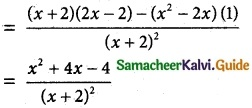Since the tangent is parallel to the x-axis.
f'(x) = 0
⇒ x² + 4x – 4 = 0
⇒ x = –$$\frac { 4±\sqrt{16+16} }{ 2 }$$
x = –$$\frac { 4±4√2 }{ 2 }$$ = -2 ± 2√2
x = -2 ± 2√2 ∈ (-1, 6)(iii) f(x) = √x – $$\frac { x }{ 3 }$$, x ∈ [0, 9]
f(0) = 0, f(9) = √9 – $$\frac { 9 }{ 3 }$$ = 3 – 3 = 0
⇒ f(0) = 0 = f(9)
f(x) is continuous on [0, 9]
f(x) is differentiable on (0, 9)
Now f'(x) = $$\frac { 1 }{ 2√x }$$ – $$\frac { 1 }{ 3 }$$
Since, the tangent is parallel to x-axis.
f'(x) = 0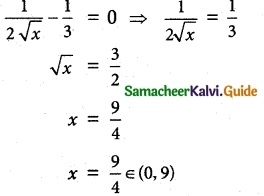Question 3.
Explain why Lagrange’s mean value theorem is not applicable to the following functions in the respective intervals:
(i) f(x) = $$\frac { 1 }{ 2√x }$$, x ∈ [-1, 2]
(ii) f(x) = |3x + 1|, x ∈ [-1, 3]
Solution:
(i) f(x) = $$\frac { 1 }{ 2√x }$$, x ∈ [-1, 2]
f(0) = undefined
∴ f(x) is not continuous at x = 0
Hence, Lagrange’s mean value theorem is not applicable.

(ii) f(x) =|3x + 1|, x ∈ [-1, 3]
The function is not differentiable at x = $$\frac{-1}{3}$$.
So Lagrange’s mean value theorem is not applicable in the given interval.

Question 4.
Using the Lagrange’s mean value theorem determine the values of x at which the tangent is parallel to the secant line at the end points of the given interval:
(i) f(x) = x³ – 3x + 2, x ∈ [-2, 2]
(ii) f(x) = (x – 2) (x – 7), x ∈ [3, 11]
Solution:
f(x) = x³ – 3x + 2, x ∈ [-2, 2]
f(x) is continuous in [- 2, 2]
f(x) is differentiable in (- 2, 2)
f(-2) = (-2)³ – 3 (-2) + 2 = – 8 + 6 + 2 = 0
f(2) = (2)³ -3(2) + 2 = 8 – 6 + 2 = 4
∴ f(x) is defined in the given interval.
Given that tangent is parallel to the secant line of the curve between x = -2 and x = 2.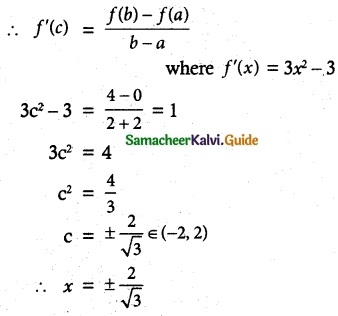(ii) f(x) = (x – 2)(x – 7), x ∈ [3, 11]
f(x) is continuous in [3, 11]
f(x) is differentiable in (3, 11)
f(3) = (3 – 2) (3 – 7) = (1) (-4) = -4
f(11) = (11 – 2) (11 – 7) = (9) (4) = 36
∴ f(x) is defined in the given interval.
Given that the tangent is parallel to the secant line ofthe curve between x = 3 and x = 11.
∴ f'(c) = $$\frac { f(b)-f(a) }{ b-a }$$
2c – 9 = $$\frac { 36+4 }{ 11-3 }$$ where f'(x) = 2x – 9
2x – 9 = $$\frac { 40 }{ 8 }$$ = 5
2c = 14 ⇒ c = 7 ∈ (3, 11)
∴ x = 7.

Question 5.
Show that the value in the conclusion of the mean value theorem for
(i) f(x) = $$\frac { 1 }{ x }$$ on a closed interval of positive numbers [a, b] is $$\sqrt { ab }$$
(ii) f(x) = Ax² + Bx + C on any interval [a, b] is $$\frac { a+b }{ 2 }$$
Solution: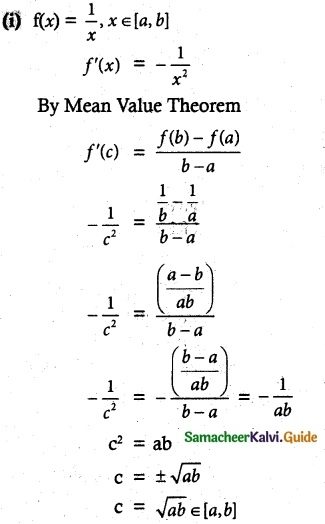(ii) f(x) = Ax² + Bx + C, x ∈ [a, b]
f'(x) = 2Ax + B
By Mean Value Theorem,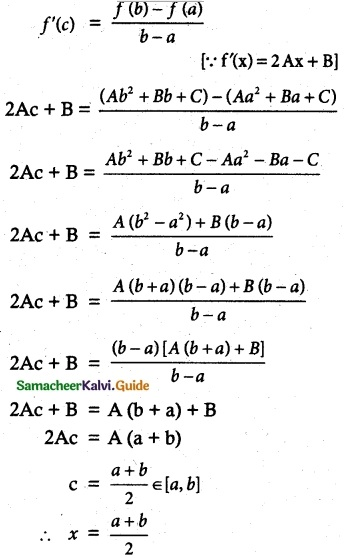Question 6.
A race car driver is racing at 20th km. If his speed never exceeds 150 km/hr, what is the maximum distance he can cover in the next two hours?
Solution:
Let a = 0, b = 2 and the interval is [0, 2] and f(0) = 20 (given)
We need to find f(2)
By Lagrange’s Mean Value Theorem,
f(b) – f(a) ≤ f'(c) [b – a]
f(b) – 20 ≤ 150(2 – 0)
f(b) ≤ 300 + 20
f(b) ≤ 320
∴ Maximum distance f(2) = 320 km.Question 7.
Suppose that for a function f(x), f'(x) ≤ 1 for all 1 ≤ x ≤ 4. Show that f(4) – f(1) ≤ 3.
Solution:
Given: For f(x), f'(x) ≤ 1 for all 1 ≤ x ≤ 4
∴ a = 1, b = 4.
By Lagrange’s Mean Value Theorem,
f(b) – f(a) ≤ f'(c) (b – a)
f(4) – f(1) ≤ 1(4 – 1)
f(4) – f(1) ≤ 3
Hence Proved.

Question 8.
Does there exist a differentiable function f(x) such that f(0) = -1, f(2) = 4 and f (x) ≤ 2 for all x. Justify your answer.
Solution:
Given:For f(x), f'(x) ≤ 2, f(0) = -1, f(2) = 4
∴ a = 0, b = 2
By Lagrange’s Mean Value Theorem,
f(b) – f(a) ≤ f'(c)(b – a)
f(2) – f(0) ≤ f'(c) (2 – 0)
$$\frac { 4+1 }{ 2 }$$ ≤ f'(c) ⇒ $$\frac { 5 }{ 2 }$$ ≤ f'(c) ≤ 2 (given)
f(x) cannot be a differentiable function in (0, 2) as f'(x) cannot be 2.5.Question 9.
Show that there lies a point on the curve f(x) = x(x + 3)e-π/2, -3 ≤ x ≤ 0 where tangent drawn is parallel to the x-axis.
Solution:
f(x) = x(x + 3)e-π/2, -3 ≤ x ≤ 0
f(x) is continuous in [-3, 0]
f(x) is differentiable in (- 3, 0)
f(-3) = -3 (-3 + 3)e-π/2 = 0
f(0) = 0
⇒ f(-3) = f(0) = 0
Since the tangent is parallel to x-axis.
f'(c) = 0
e-π/2 (2c + 3) = 0 where f'(x) = e-π/2 (2x + 3)
2c + 3 = 0
c = –$$\frac { 3 }{ 2 }$$ ∈ (-3, 0)
∴ The point lies on the curve.

Question 10.
Using Mean Value Theorem prove that for, a > 0, b > 0, |e-a – e-b| < |a – b|
Solution:
Let f(x) = e-x
f'(x) = e-x
By Lagrange’s Mean Value Theorem,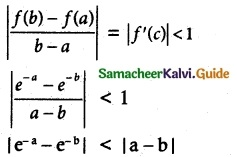Hence Proved.